# Transpose function in Microsoft Excel

In this article we will learn how we can use Transpose in Microsoft Excel.

The Transpose function is an array function which is used to rotate the contents of columns to rows and rows to columns in Microsoft Excel. Microsoft Excel has 2 options to use the Transpose Function.

Let’s take an example and understand how we can use the  Transpose  function.

I have a table in range A2:A7 which contains the Expenses for a particular department. To transpose all the contents follow the below mentioned steps:-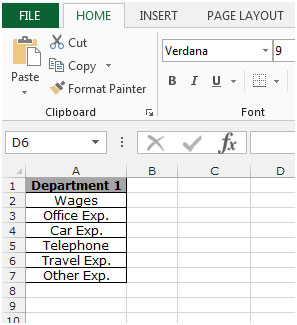Use the Transpose function with array

• Select the range B1:G1, press the key F2 on your keyboard, write the formula =Transpose (A2:A7) and press the key “CTRL+ Shift+ Enter”, The formula will show as {=Transpose (A2:A7)}
• The function will return the values in column A as headers in row 1 starting with column B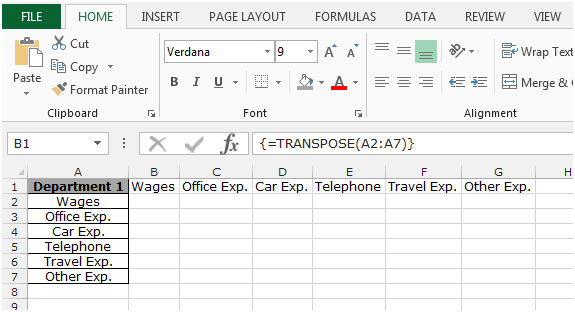• If you attempt to delete a single cell from these cells which have been transposed, it won’t be possible and an error popup will display with the message that you cannot change part of an array.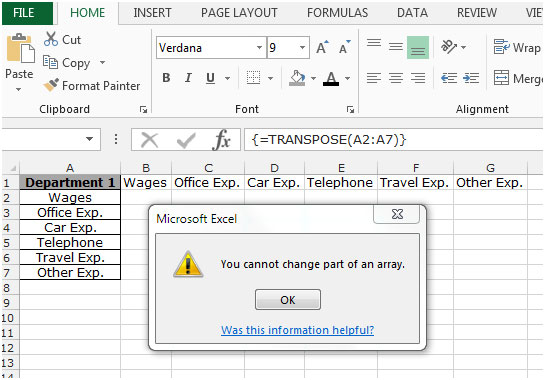Use the Transpose function as option

• Select the range A2:A7 containing the header of expenses
• Copy the content by pressing the key “CTRL + C”
• Select the cell B2, by right clickingwith the mouse and click on “Paste Special”
• The “Paste Special” dialog box  will appear  click on “Transpose” and click on OK
• The Transpose option pastes the data in the opposite direction (that is horizontally to vertically or vice versa)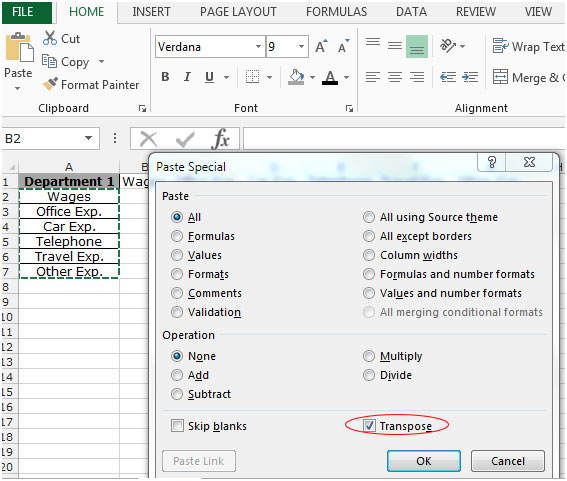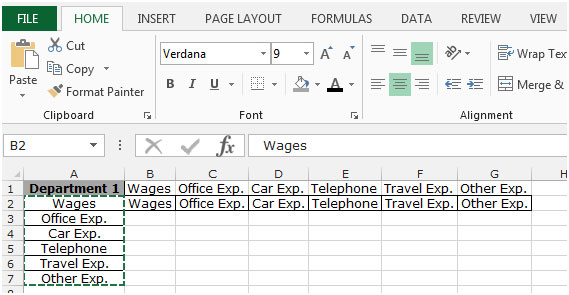This is the way  which we can use transpose function to change the direction from row to column in Microsoft Excel.

Below you can find more example to learn about Transpose:-

How to transpose cells in Microsoft Excel

How to transposing Values from Columns into Rows, and Vice Versa

How to Transpose in Microsoft Excel 2007

How to Copy Vertical and Paste Horizontal in Microsoft Excel